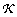# Table 1 Notation for Structural Variation (SV) statistics

Variable Meaning
Probabilistic Descriptors of SV Detection
α probability of false-positive errors
β probability of false-negative errors
Genomic and Project Parameters
λ average insert length in a Gaussian library
σ standard deviation in a Gaussian library
δ length of an instance of structural variation
τ difference threshold specified for power analysis
N number of inserts processed
G haploid subject genome length
m minimum admissible insert size (Eq. 5)
ρ haploid physical coverage (≡ N λ/G)
Labels Defining Types of SV
ISV insertion SV
DSV deletion SV
H t heterozygous SV
H m homozygous SV
Functions and Random Variables
erf Gaussian error function (see e.g. Ref. )
exp exponential functionrandom number of inserts spanning an SV site
random length of an individual insert
random mean length of inserts spanning SV site